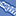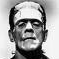# QlikView App Dev

Discussion Board for collaboration related to QlikView App Development.

Announcements
cancel
Showing results for
Did you mean:Contributor II

## Straight table - sum of rows with higher ID

Hi,

I'm having a simple table with columns ID and Value. I'm trying to find Expression for a straight table which for each line it will sum values from all others rows which have ID greater than ID of the current line.

So like

ID    Value    LookingForThis

5          10              -

4          5              10 (From ID = 5)

3          7              15 (From ID = 5 and 4)

2          6              22 (ID 5, 4 and 3)

1          5              28 (ID , 4, 3 and 2)

I hope it is clear. The real example is a bit more complicated but this is the core I believe.

I was thinking about Set Analysis like this

sum({<ID = {">CurrentID"}>} Value)

but the issue is that I don't know how to get the CurrentID in SA.

Thanks for all reactions.

Regards,

Jindra

1 Solution

Accepted SolutionsMVP

With 11.2 you are left with only 3 options

1) Sort ID in descending order in the script and use this

Aggr(RangeSum(Above(Sum(Value), 1, RowNo())), ID)

2) Do nothing in the script, but sort your chart correctly and then use this

RangeSum(Above(Sum(Value), 1, RowNo()))

3) Use The As-Of Table

8 RepliesMaster II

If your table is sorted as shown, you can use the above() function.Contributor II
Author

Tank you m w.

Unfortunately, it is usually not. There are other fields used for sorting.MVP

May be this

RangeSum(Above(Sum(Value), 1, RowNo()))Contributor II
Author

The problem is that it does not have to be always sorted like this. The higher ID rows can be also below the current line.MVP

Do you have QV12 or above? If you do, then you can do this

Aggr(RangeSum(Above(Sum(Value), 1, RowNo())), (ID, (NUMERIC, desc)))Contributor II
Author

Unfortunately on version 11.20.MVP

With 11.2 you are left with only 3 options

1) Sort ID in descending order in the script and use this

Aggr(RangeSum(Above(Sum(Value), 1, RowNo())), ID)

2) Do nothing in the script, but sort your chart correctly and then use this

RangeSum(Above(Sum(Value), 1, RowNo()))

3) Use The As-Of TableContributor II
Author

Thank you, I'm going with doing it in a script. It will restrict some possible selections for a user but will give me correct results.